Monday, 25 July 2011 09:12

## XLS to PDF in C#, VB.NET

The sample demonstrates how to convert Excel workbook to PDF file via Spire.XLS.

Get XLS-to-PDF.pdf.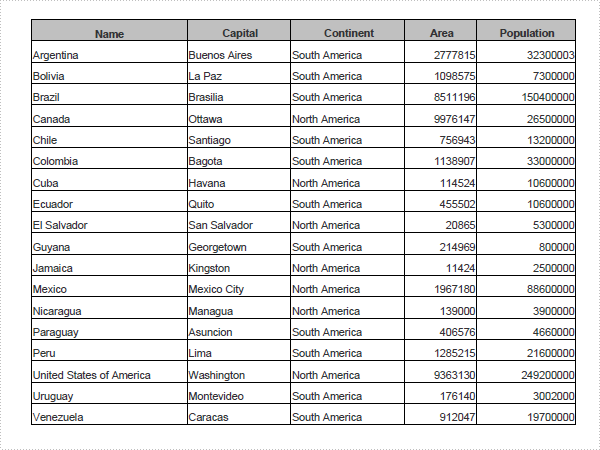Published in Misc
Monday, 18 July 2011 06:43

## How to Export Datatable to Excel through DataGridView?

Export DataTable to Excel can be very easy through Spire.DataExport (or Spire.Office). Furthermore, Spire.DataExport enables user to Export data to Excel through DataGridView. Through DataGridView users can preview and modify data information before exporting.

Download Spire.DataExport (or Spire.Office) with .NET Framework together. Only 2 Simple steps you can finish the whole datatable to Excel exporting process.

Step 1: Load Data Information

In this step, Spire.DataExport will help you load Data information from your datatable. After you put in your data source and SQL command, you can preview and modify data information in DataGridView area.

[C#]
```
private void btnLoad_Click(object sender, EventArgs e)
{
using(OleDbConnection oleDbConnection = new OleDbConnection())
{
oleDbConnection.ConnectionString = this.textBox1.Text;
OleDbCommand oleDbCommand = new OleDbCommand();
oleDbCommand.CommandText = this.textBox2.Text;
oleDbCommand.Connection = oleDbConnection;
using(OleDbDataAdapter da = new OleDbDataAdapter(oleDbCommand))
{
DataTable dt = new DataTable();
da.Fill(dt);
dataGridView1.DataSource = dt;
}
}
}

```

Effect Screenshot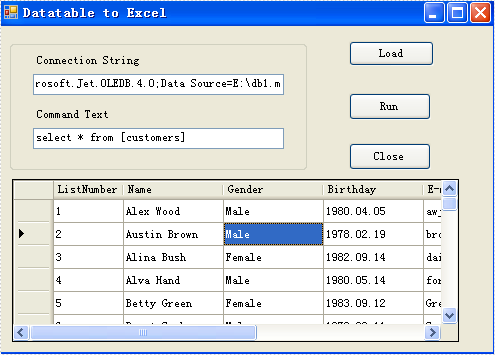Step 2: Set Export into Excel

Spire.DataExport allows user to export data into most popular file formats including MS Excel, MS Word, HTML, PDF, XML, CSV, DBF, DIF, etc. Now, in this step you should give an order to Export Data into Excel file format. Spire.DataExport will create a new MS Excel Worksheet for storing Data which exported out. You can rename the excel file in this step either.

[C#]
```        private void btnRun_Click(object sender, EventArgs e)
{
Spire.DataExport.XLS.CellExport cellExport = new Spire.DataExport.XLS.CellExport();
Spire.DataExport.XLS.WorkSheet worksheet1 = new Spire.DataExport.XLS.WorkSheet();
worksheet1.DataSource = Spire.DataExport.Common.ExportSource.DataTable;
worksheet1.DataTable = this.dataGridView1.DataSource as DataTable;
worksheet1.StartDataCol = ((System.Byte)(0));
cellExport.Sheets.Add(worksheet1);
cellExport.ActionAfterExport = Spire.DataExport.Common.ActionType.OpenView;
cellExport.SaveToFile("20110223.xls");
}

```

Effect Screenshot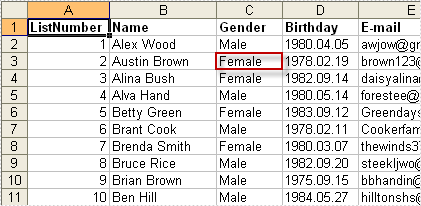Published in Datatable Export
Monday, 24 January 2011 09:49

## Save Excel Document in C#, VB.NET

Automation of an Excel file allows us to doing various operations in C#/VB.NET. Any loss in these operations may result in unexpected negative consequences for developers and the clients of the developers. That means we must find a solution that enables us to Save Excel with no loss in quality of our operations. This section will demonstrate how to fast save Excel file with perfect performance as directly operations in Excel files.

Spire.Xls for .NET is a professional component that enables developers directly manages Excel operation regardless whether Microsoft Excel is installed on or not. With Spire.Xls for .NET, we can save Excel to what we want it to be. Any kind of trial and evaluation on Spire.Xls for .NET is always welcomed; so now please feel free to download Spire.Xls for .NET and then follow our guide to save perfect Excel or try other function of Spire.Xls for .NET.

Spire.Xls for .NET allows us to create a new Excel file, write data in to it, edit the input data and then save Excel file.

[C#]
```using Spire.Xls;
namespace Excel_save
{
class Program
{
static void Main(string[] args)
{
//Create a new workbook
Workbook workbook = new Workbook();
//Initialize worksheet
Worksheet sheet = workbook.Worksheets;
//Append text
sheet.Range["A1"].Text = "Demo: Save Excel in .NET";
//Save it as Excel file
workbook.SaveToFile("Sample.xls",ExcelVersion.Version97to2003);
//Launch the file
System.Diagnostics.Process.Start(workbook.FileName);
}
}
}
```
[VB.NET]
```Imports Spire.Xls
Namespace Excel_save
Class Program
Private Shared Sub Main(args As String())
'Create a new workbook
Dim workbook As New Workbook()
'Initialize worksheet
Dim sheet As Worksheet = workbook.Worksheets(0)
'Append text
sheet.Range("A1").Text = "Demo: Save Excel in .NET"
'Save it as Excel file
workbook.SaveToFile("Sample.xls",ExcelVersion.Version97to2003)
'Launch the file
System.Diagnostics.Process.Start(workbook.FileName)
End Sub
End Class
End Namespace
```
Published in Document Operation
Monday, 24 January 2011 09:43

## Add Excel Formulas in C#, VB.NET

In an Excel Worksheet, we may import a great deal of data. Sometimes, we need to calculate the data to get other numbers we need. It is a time consuming job when we deal with large spreadsheets and more complex data. Formula is the main tool to calculate data. There are various formulas included in Excel. Below I will show you how to add formula in C#, VB.NET by Spire.XLS.

Spire.XLS .NET is a Professional and stable .NET Excel component which enables developers/programmers to operate Excel files with their ASP.NET web sites and Windows Forms applications. It supports calculate complex Excel Formulas. Spire.XLS presents you an easiest way to add formula for Excel.

Please check the effective screenshot first.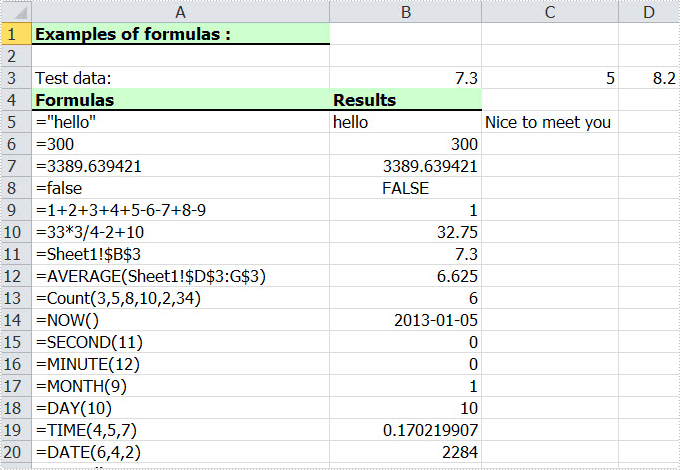Now we will give you a demo with many kinds of formulas written in the worksheet. The formula can be string, bool value, calculation, sheet area reference, time and so on. Here, we first reference a variable "currentRow" to control rows of all kinds of formulas. Second, reference "currentFormula". Assign value for Worksheet.Range [cellRange]. Text to write a formula, and then via currentFormula to assign value for Worksheet.Range [cellRange]. Formula to get the formula results.

[C#]
```using Spire.Xls;

namespace Formula
{
class Program
{
static void Main(string[] args)
{
//Create a new workbook
Workbook workbook = new Workbook();

//Initialize worksheet
Worksheet sheet = workbook.Worksheets;

//initialize currentRow
int currentRow = 3;
string currentFormula = string.Empty;

//test data
sheet.Range[currentRow, 2].NumberValue = 7.3;
sheet.Range[currentRow, 3].NumberValue = 5; ;
sheet.Range[currentRow, 4].NumberValue = 8.2;
sheet.Range[currentRow, 5].NumberValue = 4;
sheet.Range[currentRow, 6].NumberValue = 3;
sheet.Range[currentRow, 7].NumberValue = 11.3;

//string.
currentFormula = "=\"hello\"";
sheet.Range[++currentRow, 1].Text = "=\"hello\"";
sheet.Range[currentRow, 2].Formula = currentFormula;

//bool.
currentFormula = "=false";
sheet.Range[++currentRow, 1].Text = currentFormula;
sheet.Range[currentRow, 2].Formula = currentFormula;

//calculation
currentFormula = "=33*3/4-2+10";
sheet.Range[++currentRow, 1].Text = currentFormula;
sheet.Range[currentRow, 2].Formula = currentFormula;

//sheet area reference
currentFormula = "=AVERAGE(Sheet1!\$D\$3:G\$3)";
sheet.Range[++currentRow, 1].Text = currentFormula;
sheet.Range[currentRow, 2].Formula = currentFormula;

//time
currentFormula = "=NOW()";
sheet.Range[++currentRow, 1].Text = currentFormula;
sheet.Range[currentRow, 2].Formula = currentFormula;
sheet.Range[currentRow, 2].Style.NumberFormat = "yyyy-MM-DD";

//Save the file
workbook.SaveToFile("Sample.xls",ExcelVersion.Version97to2003);

//Launch the file
System.Diagnostics.Process.Start("Sample.xls");
}
}
}
```
[VB.NET]
```Imports Spire.Xls

Module Module1

Sub Main()
'Create a new workbook
Dim workbook As New Workbook()

'Initialize worksheet
Dim sheet As Worksheet = workbook.Worksheets(0)

'initialize currentRow
Dim currentRow As Integer = 3
Dim currentFormula As String = String.Empty

'test data
sheet.Range(currentRow, 2).NumberValue = 7.3
sheet.Range(currentRow, 3).NumberValue = 5

sheet.Range(currentRow, 4).NumberValue = 8.2
sheet.Range(currentRow, 5).NumberValue = 4
sheet.Range(currentRow, 6).NumberValue = 3
sheet.Range(currentRow, 7).NumberValue = 11.3

'string.
currentFormula = "=""hello"""
sheet.Range(System.Threading.Interlocked.Increment(currentRow), 1).Text = "=""hello"""
sheet.Range(currentRow, 2).Formula = currentFormula

'bool.
currentFormula = "=false"
sheet.Range(System.Threading.Interlocked.Increment(currentRow), 1).Text = currentFormula
sheet.Range(currentRow, 2).Formula = currentFormula

'calculation
currentFormula = "=33*3/4-2+10"
sheet.Range(System.Threading.Interlocked.Increment(currentRow), 1).Text = currentFormula
sheet.Range(currentRow, 2).Formula = currentFormula

'sheet area reference
currentFormula = "=AVERAGE(Sheet1!\$D\$3:G\$3)"
sheet.Range(System.Threading.Interlocked.Increment(currentRow), 1).Text = currentFormula
sheet.Range(currentRow, 2).Formula = currentFormula

'time
currentFormula = "=NOW()"
sheet.Range(System.Threading.Interlocked.Increment(currentRow), 1).Text = currentFormula
sheet.Range(currentRow, 2).Formula = currentFormula
sheet.Range(currentRow, 2).Style.NumberFormat = "yyyy-MM-DD"

'Save doc file.
workbook.SaveToFile("Sample.xls",ExcelVersion.Version97to2003)

'Launching the MS Word file.
System.Diagnostics.Process.Start("Sample.xls")

End Sub
End Module
```

Spire.XLS supports many methods to add a formula for Excel including different parts. As a professional .NET Excel component, it owns the ability of inserting content into Excel document, formatting cells and converting Excel documents to popular office file formats. Spire.XLS for WPF supports Excel 97-2003, Excel 2007 and Excel 2010.

Published in Formula
Thursday, 20 January 2011 10:33

## Insert Image in Excel in C#, VB.NET

Users are allowed to insert image in Excel files. With image, the appearance of Excel file will be more beautiful. Besides decorating, some images are related to data information in Excel. For example, the image will be a chart. These images can make readers learn data information more clearly. This guide will show an easy method to insert image in Excel with C#, VB.NET

Spire.XLS for .NET, a professional component to operating Excel files, enables users to insert image in Excel by using C# and VB.NET. This guide focuses on how to easily insert image in Excel by using Spire.XLS for .NET.  Developers can use sheet.Pictures.Add(int toprow, int leftcolumn, filename string) method to insert image in Excel directly. Below demonstrates an Excel with an image of flower.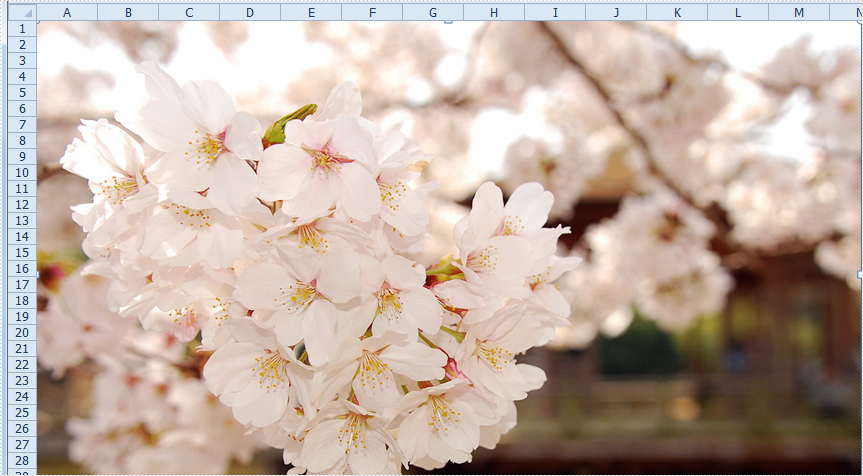Download and install Spire.XLS for .NET and then use the following code to insert image.

[C#]
```using Spire.Xls;

namespace InsertImage
{
class Program
{
static void Main(string[] args)
{
//Create Workbook
Workbook workbook = new Workbook();
Worksheet sheet = workbook.Worksheets;

//Insert Image
sheet.Pictures.Add(1, 1, @"E:\work\sample.jpg");

//Save and Launch
workbook.SaveToFile("ExcelImage.xlsx", ExcelVersion.Version2010);
System.Diagnostics.Process.Start("ExcelImage.xlsx");
}
}
}
```
[VB.NET]
```Imports Spire.Xls

Namespace InsertImage
Friend Class Program
Shared Sub Main(ByVal args() As String)
'Create Workbook
Dim workbook As New Workbook()
Dim sheet As Worksheet = workbook.Worksheets(0)

'Insert Image
sheet.Pictures.Add(1, 1, "E:\work\sample.jpg")

'Save and Launch
workbook.SaveToFile("ExcelImage.xlsx", ExcelVersion.Version2010)
System.Diagnostics.Process.Start("ExcelImage.xlsx")
End Sub
End Class
End Namespace
```
Published in Image
Thursday, 20 January 2011 10:26

## Create Excel Pie Chart in C#, VB.NET

### Why We Create Excel Pie Charts?

Create excel pie charts is a simple method to display your data to other individuals or a group. Excel pie charts can easily relay your messages that may otherwise go unnoticed by your audience. Pie charts are helpful for international audiences because they are universally known and easily explained. And it's extremely easy to create and use in Excel.

Pie charts, unlike other charts in Microsoft Excel, require the data in your worksheet be contained in only one row or column. By indicating a "category", an additional row or column can be used. Relative sizes are easy to compare as each piece of data is represented as a portion of a whole.

### How to Create Excel Pie Charts in Microsoft Excel?

Every one of you can create excel pie charts in no time by using Microsoft Excel through the following steps:

• Launch Microsoft Office Excel and open your excel file which with the data that you want to base your pie chart on.
• Select the data that you want to base your chart on. The last cell that you want to select will not be chosen but it will have bold outline borders around it.
• Click "Insert" button to open Chart Wizard window. Click on "Pie" in the right side column of Chart Type. Several sub-types of pie charts will be offered for you to choose. Pick a proper type which can best match with your data and click "Next"
• Prevew on this new interface. If the pie chart with wrong information or even no pie chart appears, click "Cancel" and select your data, try the above process again. If everything is OK, click "Next"
• On the new window, enter labels and a title for your pie chart. Click "Finish" when you see the pie chart appears as you want.
• On your spreadsheet, click the box and drag your mouse to manipulate the size of the chart. By right clicking the chart and box, you can get many options to edit the pie chart.

### How to Use Spire.XLS for .NET to Creat Excel Pie Charts?

Spire.XLS presents you an easy way to create a pie chart in the Excel workbook. First, you should create a pie chart with the sheet.Charts.Add method. You may control the resource of the data and title of the chart by setting DataRange and ChartTitle properties. What's more, we create an object to operate more about the chart. You may set the label and value of the pie with the properties cs.CategoryLabels and cs.Values. If you want to hide the value of the pie, you may set the cs.DataPoints.DefaultDataPoint.DataLabels.HasValue property false. In this demo, in order to reflect the effect of the chart, we set the grid lines of the worksheet invisible by assigning the sheet.GridLinesVisible false.

Use the C#/VB.NET codes of Spire.XLS for .NET below to create excel pie charts:

[C#]
```using Spire.Xls;
using System.Drawing;

namespace Saveas
{
class Program
{
static void Main(string[] args)
{
//Create a new workbook
Workbook workbook = new Workbook();

//Initialize worksheet
workbook.CreateEmptySheets(1);
Worksheet sheet = workbook.Worksheets;

//Set sheet name
sheet.Name = "Chart data";

//Set the grid lines invisible
sheet.GridLinesVisible = false;

//Create a chart
Chart chart = sheet.Charts.Add(ExcelChartType.Pie3D);

//Set region of chart data
chart.DataRange = sheet.Range["B2:B5"];
chart.SeriesDataFromRange = false;

//Set position of chart
chart.LeftColumn = 1;
chart.TopRow = 6;
chart.RightColumn = 9;
chart.BottomRow = 25;

//Chart title
chart.ChartTitle = "Sales by year";
chart.ChartTitleArea.IsBold = true;
chart.ChartTitleArea.Size = 12;

//Initialize the chart series
Spire.Xls.Charts.ChartSerie cs = chart.Series;

//Chart Labels resource
cs.CategoryLabels = sheet.Range["A2:A5"];

//Chart value resource
cs.Values = sheet.Range["B2:B5"];

//Set the value visible in the chart
cs.DataPoints.DefaultDataPoint.DataLabels.HasValue = true;

//Year
sheet.Range["A1"].Value = "Year";
sheet.Range["A2"].Value = "2002";
sheet.Range["A3"].Value = "2003";
sheet.Range["A4"].Value = "2004";
sheet.Range["A5"].Value = "2005";

//Sales
sheet.Range["B1"].Value = "Sales";
sheet.Range["B2"].NumberValue = 4000;
sheet.Range["B3"].NumberValue = 6000;
sheet.Range["B4"].NumberValue = 7000;
sheet.Range["B5"].NumberValue = 8500;

//Style
sheet.Range["A1:B1"].Style.Font.IsBold = true;
sheet.Range["A2:B2"].Style.KnownColor = ExcelColors.LightYellow;
sheet.Range["A3:B3"].Style.KnownColor = ExcelColors.LightGreen1;
sheet.Range["A4:B4"].Style.KnownColor = ExcelColors.LightOrange;
sheet.Range["A5:B5"].Style.KnownColor = ExcelColors.LightTurquoise;

//Border
sheet.Range["A1:B5"].Style.Borders[BordersLineType.EdgeTop].Color = Color.FromArgb(0, 0, 128);
sheet.Range["A1:B5"].Style.Borders[BordersLineType.EdgeTop].LineStyle = LineStyleType.Thin;
sheet.Range["A1:B5"].Style.Borders[BordersLineType.EdgeBottom].Color = Color.FromArgb(0, 0, 128);
sheet.Range["A1:B5"].Style.Borders[BordersLineType.EdgeBottom].LineStyle = LineStyleType.Thin;
sheet.Range["A1:B5"].Style.Borders[BordersLineType.EdgeLeft].Color = Color.FromArgb(0, 0, 128);
sheet.Range["A1:B5"].Style.Borders[BordersLineType.EdgeLeft].LineStyle = LineStyleType.Thin;
sheet.Range["A1:B5"].Style.Borders[BordersLineType.EdgeRight].Color = Color.FromArgb(0, 0, 128);
sheet.Range["A1:B5"].Style.Borders[BordersLineType.EdgeRight].LineStyle = LineStyleType.Thin;

//Number format
sheet.Range["B2:C5"].Style.NumberFormat = "\"\$\"#,##0";
chart.PlotArea.Fill.Visible = false;

//Save the file
workbook.SaveToFile("Sample.xls",ExcelVersion.Version97to2003);

//Launch the file
System.Diagnostics.Process.Start("Sample.xls");
}
}
```
[VB.NET]
```Imports Spire.Xls
Imports System.Drawing

Module Module1

Sub Main()
'Create a new workbook
Dim workbook As New Workbook()

'Initialize worksheet
workbook.CreateEmptySheets(1)
Dim sheet As Worksheet = workbook.Worksheets(0)

'Set sheet name
sheet.Name = "Chart data"

'Set the grid lines invisible
sheet.GridLinesVisible = False

'Create a chart
Dim chart As Chart = sheet.Charts.Add(ExcelChartType.Pie3D)

'Set region of chart data
chart.DataRange = sheet.Range("B2:B5")
chart.SeriesDataFromRange = False

'Set position of chart
chart.LeftColumn = 1
chart.TopRow = 6
chart.RightColumn = 9
chart.BottomRow = 25

'Chart title
chart.ChartTitle = "Sales by year"
chart.ChartTitleArea.IsBold = True
chart.ChartTitleArea.Size = 12

'Set the chart
Dim cs As Spire.Xls.Charts.ChartSerie = chart.Series(0)

'Chart Labels resource
cs.CategoryLabels = sheet.Range("A2:A5")

'Chart value resource
cs.Values = sheet.Range("B2:B5")

'Set the value visible in the chart
cs.DataPoints.DefaultDataPoint.DataLabels.HasValue = True

'Year
sheet.Range("A1").Value = "Year"
sheet.Range("A2").Value = "2002"
sheet.Range("A3").Value = "2003"
sheet.Range("A4").Value = "2004"
sheet.Range("A5").Value = "2005"

'Sales
sheet.Range("B1").Value = "Sales"
sheet.Range("B2").NumberValue = 4000
sheet.Range("B3").NumberValue = 6000
sheet.Range("B4").NumberValue = 7000
sheet.Range("B5").NumberValue = 8500

'Style
sheet.Range("A1:B1").Style.Font.IsBold = True
sheet.Range("A2:B2").Style.KnownColor = ExcelColors.LightYellow
sheet.Range("A3:B3").Style.KnownColor = ExcelColors.LightGreen1
sheet.Range("A4:B4").Style.KnownColor = ExcelColors.LightOrange
sheet.Range("A5:B5").Style.KnownColor = ExcelColors.LightTurquoise

'Border
sheet.Range("A1:B5").Style.Borders(BordersLineType.EdgeTop).Color = Color.FromArgb(0, 0, 128)
sheet.Range("A1:B5").Style.Borders(BordersLineType.EdgeTop).LineStyle = LineStyleType.Thin
sheet.Range("A1:B5").Style.Borders(BordersLineType.EdgeBottom).Color = Color.FromArgb(0, 0, 128)
sheet.Range("A1:B5").Style.Borders(BordersLineType.EdgeBottom).LineStyle = LineStyleType.Thin
sheet.Range("A1:B5").Style.Borders(BordersLineType.EdgeLeft).Color = Color.FromArgb(0, 0, 128)
sheet.Range("A1:B5").Style.Borders(BordersLineType.EdgeLeft).LineStyle = LineStyleType.Thin
sheet.Range("A1:B5").Style.Borders(BordersLineType.EdgeRight).Color = Color.FromArgb(0, 0, 128)
sheet.Range("A1:B5").Style.Borders(BordersLineType.EdgeRight).LineStyle = LineStyleType.Thin

'Number format
sheet.Range("B2:C5").Style.NumberFormat = """\$""#,##0"
chart.PlotArea.Fill.Visible = False

'Save doc file.
workbook.SaveToFile("Sample.xls",ExcelVersion.Version97to2003)

'Launch the MS Word file.
System.Diagnostics.Process.Start("Sample.xls")

End Sub
End Module
```

After running the demo, you may find a pie appear in the document: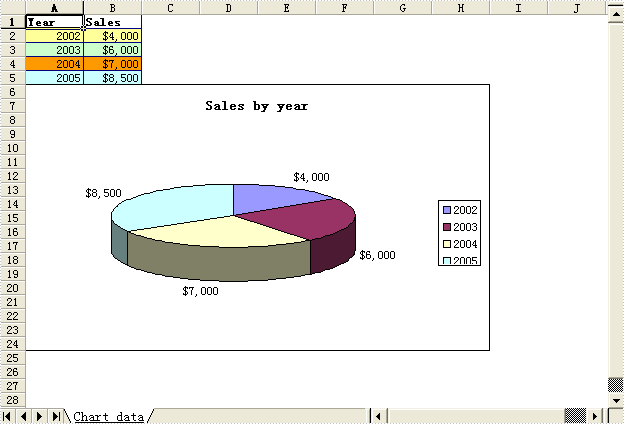Published in Chart
Thursday, 01 July 2010 14:22

## EXCEL Interior in C#, VB.NET

The sample demonstrates how to write excel 2007 workbook.Published in Excel2007
Saturday, 03 July 2010 10:17

## EXCEL Pagesetup in C#, VB.NET

The sample demonstrates how to work with page setup in an excel workbook.Published in Page Setup
Saturday, 03 July 2010 10:09

## EXCEL DataValidation in C#, VB.NET

The sample demonstrates how to write validation into spreadsheet.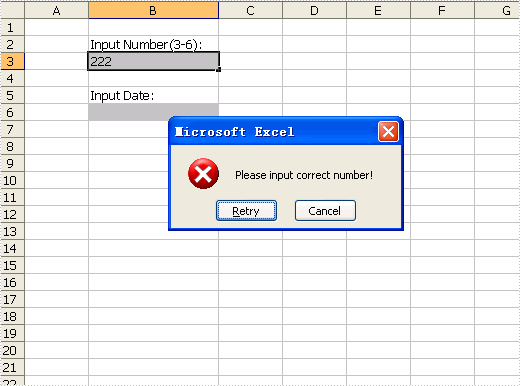Published in Validation
Saturday, 03 July 2010 10:05

## EXCEL Write Filters in C#, VB.NET

The sample demonstrates how to create auto filters in an excel workbook.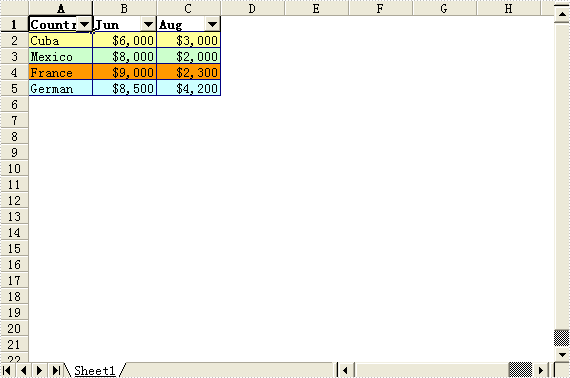Published in Filters
Page 1 of 3